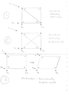# Kinematic indeterminancy

• rmrramani

#### rmrramani

can u explain what is kinematic indeterminancy?

I have never even heard this term, but I would be interested to know the context in which you heard it.

its similar to static indeterminancy,i red in (that word only i got)TH.megson(structural and stress analysis)

It's a structural engineering term.

Look at the attached sketches.

(1) This situation is called Statically Determinate.

Shows a simple pin jointed truss with 5 members.
It is supported at A and B and loaded in some way at D
It is also restrained from sideways movement at A, or load L would just push it sideways.

This is a structure because it does not change shape in response to load L. It remains a rectangle with one diagonal.

Since there are just enough equilibrium equations (horizontal, vertical and moments) we can completely determined the forces in the members, given L and the dimensions.

(2) This situation is called Statically Indeterminate.

Shows the same thing with one extra diagonal member added.
A little calculation will show that we no longer have enough equations to uniquely determine the member forces, although the reactions will not have changed.

(3) This situation is called Kinematically Indeterminate.

The same rectangle, but we have taken away both diagonals. This is no longer a structure it is now a mechanism because it can (and will) change shape to a parallelogram in response to load L.
Although it remains fixed at A and B, it is not possible to determine how far the rectangle collapse from the equations of equilibrium alone. So the situation is indeterminate and the member forces cannot be calculated from equilibrium.

#### Attachments

•mech.jpg
10.6 KB · Views: 377
Studiot, thank you for this reply. It is both concise and precise.